International Journal of Accounting, Finance and Risk Management
Volume 2, Issue 1, February 2017, Pages: 21-30

Portfolio Optimization Using Matrix Approach: A Case of Some Stocks on the Ghana Stock Exchange

Abonongo John1, Anuwoje Ida Logubayom2, *, Ackora-Prah J.1

1College of Science, Department of Mathematics, Kwame Nkrumah University of Science and Technology, Kumasi, Ghana

2Faculty of Mathematical Sciences, Department of Statistics, University for Development Studies, Navrongo, Ghana(A. I. Logubayom)

*Corresponding author

Abonongo John, Anuwoje Ida Logubayom, Ackora-Prah J. Portfolio Optimization Using Matrix Approach: A Case of Some Stocks on the Ghana Stock Exchange. International Journal of Accounting, Finance and Risk Management. Vol. 2, No. 1, 2017, pp. 21-30. doi: 10.11648/j.ijafrm.20170201.14

Received: September 11, 2016; Accepted: November 25, 2016; Published: February 13, 2017

Abstract: Analyzing risk has been a principal concern of actuarial and insurance professionals which plays a fundamental role in the theory of portfolio selection where the prime objective is to find a portfolio that maximizes expected return while reducing risk. Portfolio optimization has been applied to asset management and in building strategic asset allocation. The purpose of this paper is to construct optimal and efficient portfolios using the matrix approach. This paper used secondary data on 13 stocks (ETI, GCB, GOIL, TOTAL, FML, GGBL, CLYD, EGL, PZC, UNIL, TLW, AGA and BOPP) from the Ghana Stock Exchange (GSE) database comprising the monthly closing prices from the period 02/01/2004 to 16/01/2015. The results revealed that, all the portfolios were optimal and that portfolios 1, 2, 4, 5, 6, 9, 10, 11 and 12 with expected return 2.523, 2.593, 2.827, 3.642, 2.405, 2.812, 5.229, 3.559 and 5.928 respectively were efficient portfolios whereas portfolios 3, 7 and 8 with expected return 0.377, 0.699 and 0.152 respectively were inefficient portfolios with reference to the expected return of the global minimum variance portfolio (2.360). GGBL was seen as the stock with the highest allocation of wealth in most of the portfolios. Six out of the 12 portfolios had CLYD exhibiting the least asset allocation.

Keywords: Portfolio Optimization, Efficient Frontier, Mean-Variance, Matrix Approach

1. Introduction

After Markowitz ground-breaking work in portfolio selection Markowitz  portfolio optimization has been receiving greater attention from asset and liability managers, academics and risk managers. Most of the studies explain a portfolio optimization criterion such as mean-variance, conditional value-at-risk, value-at-risk, mean absolute deviation, stochastic dominance of first and second order among others.

The mean-variance is the traditional optimization approach introduced by Markowitz. But before Markowitz presented his approach, portfolio theory was a relevant area of research. However, the main focus of Bachelier and his successor was to improve performance. Markowitz focused on risk. He established volatility as a major risk measure in portfolio theory and showed how the risk can be reduced by diversification. He demonstrated how financial portfolios which have max expected return for a given risk level can be estimated.

In portfolio analysis, variance measures the volatility (risk) of an asset or group of assets, hence larger variance indicates greater risk and vice versa. When many assets are held together in a portfolio, assets decreasing in value are usually offset by portfolios asset increasing in value, hence minimizing risk. Also, the total variance of a portfolio is usually lower than a simple weighted average of the individual asset variances . The return of any financial asset is described by a random variable, whose expected mean and variance are assumed to be reliably estimated from historical data. The expected mean and variance are interpreted as the compensation and the risk respectively. The portfolio optimization problem can be formulated as follows: given a set of assets, characterized by their returns and covariance, find the optimal weight of the asset such that the overall portfolio provides the lowest risk for a given overall return. This problem reduces to find the efficient frontier, which is the set of all achievable (attainable) portfolios that offers a higher return for a given risk level. When the number of assets in a portfolio becomes large, the total variance is actually derived from the covariance than from the variances of the assets .

The theory of portfolio optimization is generally associated with the classical mean-variance optimization framework of Markowitz . The drawback of the mean-variance analysis is mainly related to its sensitivity to the estimation error of the means and covariance matrix estimation of the returns of the asset. Also, it is argued that estimates of the covariance matrix are more accurate than those of the expected returns (, ). Several studies concentrates on improving the performance of the global minimum-variance portfolio (GMVP), which provides the least possible portfolio risk and involves only the covariance matrix estimates.

The classical mean-variance framework depends on the perfect knowledge of the expected returns of the assets and their variance-covariance matrix. However, these returns are unobservable and unknown. The impossibility to obtain a sufficient number of data samples, instability of data, differing personal views of decision makers on the future returns  affect their estimation and has led to what  call estimation risk in portfolio selection. This estimation risk has shown to be the source of very erroneous decisions, for, as pointed in (, ), the composition of the optimal portfolio is very sensitive to the mean and the covariance matrix of the asset returns and agitation in the moments of the random returns can result in the difficulties in constructing different optimization.

, examined portfolio optimization with correlation matrix. The results showed how to perform portfolio optimizations using mean-correlation instead of mean-variance analysis and that the two alternatives set-up produced equivalent optimization weights if correlation-based number transformed back to mean-variance ones. Also, the analysis, presented strengthens the role of regression methods in portfolio analysis. , presented a simplified perspective of Markowitz contributions to Modern Portfolio Theory (MPT), foregoing in-depth presentation of the complex mathematical/statistical models typically associated with discussions of this theory and suggested efficient computer-based ‘short cuts’.

Also, (, , ) have studied the mean variance framework in a robust context, assuming that the expected return is stochastic. They characterize the parameters involved in the mean and the variance-covariance matrix with specific types of uncertainty, and built semi-definite or second-order cone programs.

On efficient and optimal portfolios,  stated that portfolios are efficient when they provide the maximum possible expected return for a certain risk level. When building efficient portfolio one need to assume that investors are risk-averse, meaning that they will choose the portfolio with the least risk. When faced with several portfolios with the same expected return, but with different risk levels. Also, a risk-averse investor will choose the portfolio with the highest return, when they have to choose from a set of portfolios with the same risk, but different expected returns. This indicates that efficient portfolios are located in the efficient frontier (minimum-variance frontier). An optimal portfolio is one that has the minimum risk for a given level of return and an efficient portfolio is one that has the maximum expected rerun for a given level of risk. Thus, all portfolios on the minimum-variance frontier are optimal, but only those in the upper portion-at above the global minimum-variance portfolio are efficient.

The purpose of this paper is to construct optimal and efficient portfolios using matrix approach. This will give investors an insight in diversification, asset management and risk management. It will also aid investors and academics on how to construct optimal and efficient portfolios using matrix approach.

2. Materials and Methods

2.1. Source of Data

This paper used secondary data of 13 stocks (ETI, GCB, GOIL, TOTAL, FML, GGBL, CLYD, EGL, PZC, UNIL, TLW, AGA and BOPP) from the Ghana Stock Exchange (GSE) database comprising the daily closing prices from the period 02/01/2004 to 16/01/2015.

2.2. Methods of Data Analysis

The daily index series were converted into compound returns given by;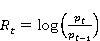(1)

where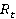is the continuous compound returns at time,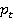is the current closing stock price index at timeand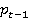is the previous closing stock price index. These returns were converted into monthly returns by assuming 365 days a year and averaging to get 30 days a month. This was then multiplied by the daily returns to obtain the monthly returns. The same method was employed in obtaining the monthly standard deviations by multiplying the square root of 30 by the daily standard deviations.

For an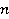-asset portfolio problem with assets given by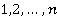. Letdenote the return on assetwith a constant expected return model given by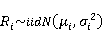(2)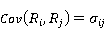(3)

Assuming that all wealth in the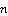-asset is given by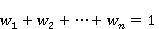(4)

Then, the portfolio return,is given by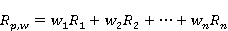(5)

where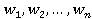are the weights of the portfolio and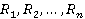are the returns of the individual stocks.

From Equation 5, the expected return on the portfolio is given by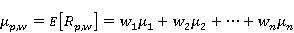(6)

and the variance of the portfolio return given by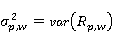(7)

2.3. Portfolio Characteristics Using Matrix Approach

The asset returns and portfolio weights are given by an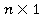column vector;(8)(9)

The probability distribution of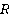is the joint distribution of the elements of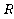. In the constant expected model, all returns are jointly normally distributed and is characterized by the mean, variance and covariance of the returns. Applying matrix notation, the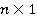vector of portfolio expected return is given by(10)

and the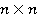covariance matrix of returns;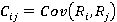is given by(11)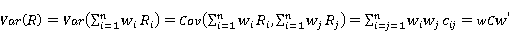(12)

Also, the condition that the portfolio weights sum to one (1) is given by(13)

where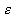is anvector with entries equal 1.

2.4. Estimating the Global Minimum Variance Portfolio

The global minimum-variance portfolio is simply the portfolio on the efficient frontier that has the least risk. It is given by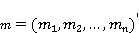(14)

where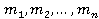are the global minimum-variance portfolio weights for asset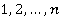.

For an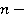asset case, the constrained minimization problem is given by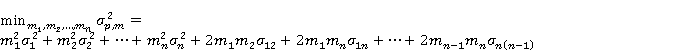(15)

Thus,(16)

Also, the first order linear equation is given by(17)

Therefore,(18)

Equation (18) is of the form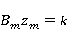(19)

where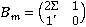,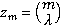and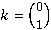Thus, solving for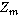from Equation (19), we get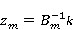(20)

where the elements of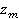are the portfolio weights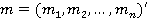for the global minimum variance portfolio return,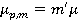and variance,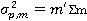.

2.5. Determining the Efficient Portfolios

For an n-asset case, the investment opportunity set in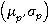-space is explained by set of values whose shape depends on the covariance terms. Assuming that investors select portfolios that maximizes expected return subject to a target level of risk or minimize risk subject to a target expected return, the asset allocation problem can be streamlined by only concentrating on the set of efficient portfolios. These portfolios lie on the boundary of the investment opportunity set above the global minimum variance portfolio.

Following Markowitz , we assume that investors wish to find portfolios that have the best expected return-risk trade off. Firstly, investors wish to find portfolios that maximizes portfolio expected return for a given risk level as measured by portfolio variance or standard deviation. This constrained maximization problem to find an efficient portfolio is given by(21)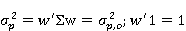(22)

Markowitz also showed that the investor’s problem of maximizing portfolio expected return subject to a target risk level has a correspondent dual representation in which the investor minimizes the risk of the portfolio subject to a given expected return. This dual problem is the constrained minimization problem which is given by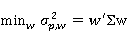(23)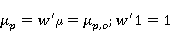(24)

In this paper, the dual problem is considered due to computational convenience and that investors being more willing to specify target expected returns rather than risk.

In solving the constrained minimization problem in Equations (23 and 24), the Lagrangian function is employed and is given by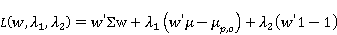(25)

where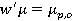and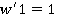are the two constraints and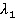and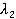are the two Lagrangian multipliers.

The first order conditions for a minimum are given by the following linear equations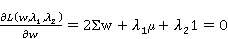(26)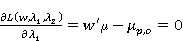(27)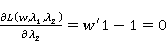(28)

Also, representing the system of linear equations in matrix form, we get(29)

Equation (29) is of the form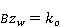(30)

where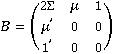,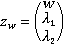and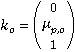Solving for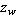, we get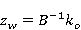(31)

If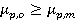then portfolio,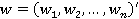is an efficient portfolio otherwise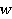is an inefficient portfolio. Also, all portfolios on the minimum variance frontier are optimal, but only those in the upper portion (at or above) the global minimum-variance portfolio are efficient.

3. Results and Discussion

Table 1 shows the descriptive statistics of the stocks. With much emphasis on the monthly returns and standard deviations, the results show that, the monthly expected return ranges from -0.547 to 5.928 with the highest return found in BOPP and the least return found in CLYD. All the stocks made gains (positive expected return) with the exception of CLYD which made a loss (negative expected return). The monthly standard deviation (risk) ranged from 8.498 to 35.547 with ETI (35.547) been the stock with the highest risk level compared with GGBL (8.498) which had the least risk level. Even though, the highest mean return was found in BOPP, its risk level was less than that of ETI. This implies that, ETI is much riskier than the rest of the stocks and that an investors need to reduce this risk by diversifying. For risk averse investors, it will be prudent to go in for GGBL since it had the least risk level compared to the rest of the stocks.

Table 1. Descriptive Statistics.

 Stock Daily Expected Return Monthly Expected return Daily Std. Dev Monthly Std. Dev ETI 0.083 2.523 6.463 35.547 GCB 0.085 2.593 1.926 10.594 GOIL 0.012 0.377 2.097 11.536 TOTAL 0.093 2.827 4.328 23.804 FML 0.120 3.642 2.157 11.864 GGBL 0.079 2.405 1.545 8.498 CLYD -0.018 -0.547 4.598 25.289 EGL 0.023 0.699 3.798 20.889 PZC 0.005 0.152 3.165 17.408 UNIL 0.093 2.812 1.882 10.352 TLW 0.172 5.229 5.270 28.985 AGA 0.117 3.557 3.346 18.403 BOPP 0.195 5.928 4.196 23.078

Table 2 shows the covariance matrix of the stocks. This provides a first-hand information on how the returns move together in a whole.

Table 2. Covariance Matrix of the Stocks.

 Stock ETI GCB GOIL TOTAL FML GGBL CLYD EGL PZC UNIL TLW AGA BOPP ETI 41.770 -2.013 -1.231 -2.284 1.047 0.207 0.362 4.116 -0.910 -1.550 0.355 -0.284 1.350 GCB -2.013 3.710 -0.047 1.615 -0.479 -0.188 0.027 -2.964 0.426 1.147 -0.095 0.852 -1.269 GOIL -1.231 -0.047 4.399 -0.166 -0.229 0.412 0.025 0.749 -0.042 -0.015 0.144 -0.015 0.111 TOTAL -2.284 1.615 -0.166 18.734 -0.646 -0.458 -1.379 -3.483 0.408 1.308 0.020 0.127 -0.174 FML 1.047 -0.479 -0.229 -0.646 4.653 0.799 1.455 1.732 -0.572 -0.545 -0.179 0.757 1.650 GGBL 0.207 -0.188 0.412 -0.458 0.799 2.387 0.446 2.006 0.128 -0.618 0.053 0.642 1.221 CLYD 0.362 0.027 0.025 -1.379 1.455 0.446 21.138 -0.144 0.394 -0.016 -0.425 -0.243 1.232 EGL 4.116 -2.964 0.749 -3.483 1.732 2.006 -0.144 14.427 -1.230 -2.382 0.174 -0.217 3.298 PZC -0.910 0.426 -0.042 0.408 -0.572 0.128 0.394 -1.230 10.017 1.229 -0.042 0.205 0.168 UNIL -1.550 1.147 -0.015 1.308 -0.545 -0.618 -0.016 -2.382 1.229 3.542 0.188 0.347 -1.635 TLW 0.355 -0.095 0.144 0.020 -0.179 0.053 -0.425 0.174 -0.042 0.188 27.777 -0.005 -0.504 AGA -0.284 0.852 -0.015 0.127 0.757 0.642 -0.243 -0.217 0.205 0.347 -0.005 11.199 -0.328 BOPP 1.350 -1.269 0.111 -0.174 1.650 1.221 1.232 3.298 0.168 -1.635 -0.504 -0.328 17.603

Figure 1 shows the monthly plot of risk-return of the stocks. The plot shows that, GOIL, PZC and EGL recorded low returns with higher risk levels compared with FML, AGA, BOPP and TLW which recorded higher returns with somewhat lower risk levels. ETI recorded the highest risk level of 35.547 with an expected return of 2.523. GGBL recorded the least risk with an expected return of 8.498. Since investors are only interested in forming optimal and efficient portfolios, CLYD was not considered since it made a loss. Also, the risk-return plot indicates that, equally weighted portfolio has higher expected return per the level of risk.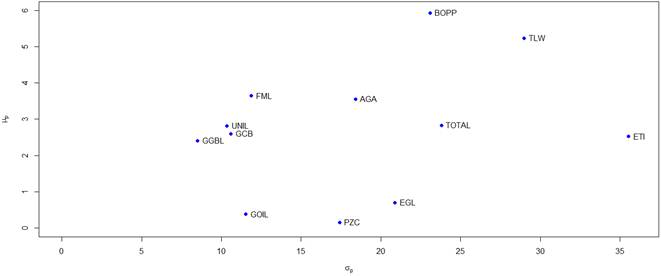Figure 1. Monthly plot of the risk and return of the stocks.

Table 3, shows the estimates of the global minimum-variance portfolio. The results reveals that, the expected return on the portfolio called global minimum-variance is 2.360 and a risk level of 0.766. This means that, there is a 0.766 risk in investing in the minimum-variance portfolio that rewards 2.360. The global minimum-variance portfolio has portfolio weights (asset allocation) as follows;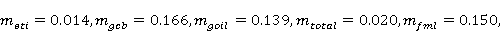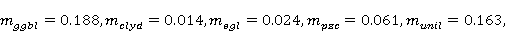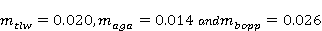.

In a vector form, the allocation of assets for the global minimum-variance portfolio is given by;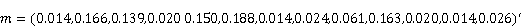(32)

In other to achieve this return, an investor needs to allocate assets given Equation 32 for investing in the portfolio with the least risk.

Table 3. Global Minimum Variance Portfolio.

 Stock Global Minimum Variance portfolio Weight Expected Return (μp,m) Portfolio Std. dev (σp,m) ETI 0.014 2.360 0.766 GCB 0.166 GOIL 0.139 TOTAL 0.020 FML 0.150 GGBL 0.188 CLYD 0.014 EGL 0.024 PZC 0.061 UNIL 0.163 TLW 0.020 AGA 0.014 BOPP 0.026

Table 4, shows the efficient portfolio with the same expected return as a given stock. The results indicate that, when ETI, GCB, GOIL, TOTAL, FML, GGBL, EGL, PZC, UNIL, TLW, AGA and BOPP with expected returns 2.523, 2.593, 0.377, 2.827, 3.642, 2.405, 0.699, 0.152, 2.812, 5.229, 3.559 and 5.928 respectively, then in other to have a portfolio whose expected return will be the same as that of as any of the above assets, an investor need to bear a risk 0.771, 0.776, 1.288, 0.805, 1.019, 0.067, 1.157, 1.384, 0.802, 1.685, 0.990 and 2.017 for holding portfolio 1, 2,…,12 respectively which are all lesser than the individual risk associated with the assets. This indicates that, one reduces risk by diversifying in several assets that are uncorrelated. In portfolio 1, 2, …, 12, the highest proportion of asset allocation is found in GGBL (0.188), GGBL (0.189), GGBL (0.188), GOIL (0.382), GGBL (0.190), GGBL (0.194), GGBL(0.179), PZC (0.192), GGBL(0.190), FML (0.356), FML (0.236) and FML (0.406) respectively. GGBL is seen as the stock with the highest allocation of wealth in most of the portfolios. This is so because from Table 1, GGBL exhibited the least standard deviation (risk) and since investors are interested in minimizing risk given a target expected return, hence much wealth allocation in GGBL. The least allocation of wealth in portfolio 1, 2, …, 12 was CLYD (0.007), CLYD (0.004), BOPP (-0.102), CLYD (-0.005), CLYD (-0.040), CLYD (0.012), BOPP (-0.081), BOPP (-0.116), CLYD (-0.005), GOIL (-0.212), EGL (-0.177) and GOIL (-0.297) respectively. Six out of the 12 portfolios had CLYD exhibiting the least asset allocation. This is because even though from Table 1, CLYD had a higher risk level but no compensation for holding it since it made a loss. For an investor to adequately minimize risk in other to achieve the expected return in each portfolio, the proportion of wealth to be allocated to each asset in each portfolio is given by the following vectors;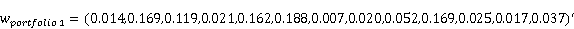(33)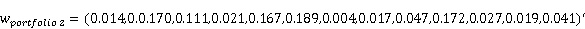(34)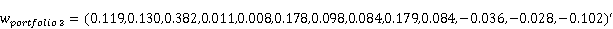(35)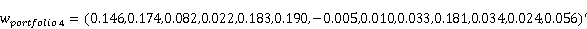(36)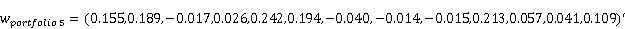(37)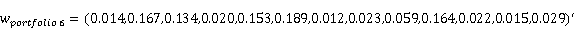(38)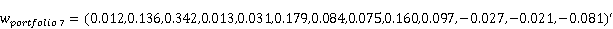(39)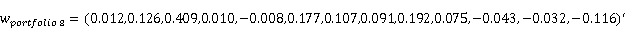(40)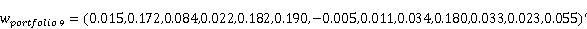(41)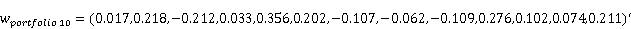(42)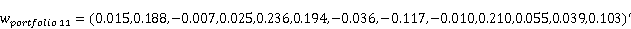(43)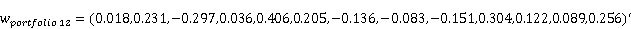(44)

The efficient portfolios were selected by taking into consideration the expected return of each portfolio. That is, any portfolio with expected return greater or equal the expected return of the global minimum-variance portfolio is considered efficient portfolio otherwise the portfolio is an inefficient one. From the results, portfolios 1, 2, 4, 5, 6, 9, 10, 11 and 12 with expected return 2.523, 2.593, 2.827, 3.642, 2.405, 2.812, 5.229, 3.559 and 5.928 respectively are considered efficient portfolios since their expected return each is greater than the expected return of the global minimum variance portfolio. This indicates that, these portfolios have maximum expected for the level risk estimated. Portfolios 3, 7 and 8 with expected return 0.377, 0.699 and 0.152 respectively are considered inefficient portfolios since their expected each is less than the expected return of the global minimum-variance portfolio.

Table 4. Efficient Portfolio with the same expected return as a given stock.

 Portfolio No. Stock Stock Expected Return Weight Portfolio Expected Return (μp,o) Portfolio Std. dev (σp,o) 1 ETI* 2.523 0.014 2.523 0.771 GCB 0.169 GOIL 0.119 TOTAL 0.021 FML 0.162 GGBL 0.188 CLYD 0.007 EGL 0.020 PZC 0.052 UNIL 0.169 TLW 0.025 AGA 0.017 BOPP 0.037 ETI 0.014 2 GCB* 2.593 0.170 2.593 0.776 GOIL 0.111 TOTAL 0.021 FML 0.167 GGBL 0.189 CLYD 0.004 EGL 0.017 PZC 0.047 UNIL 0.172 TLW 0.027 AGA 0.019 BOPP 0.041 ETI 0.119 GCB 0.130 3 GOIL* 0.377 0.382 0.377 1.288 TOTAL 0.011 FML 0.008 GGBL 0.178 CLYD 0.098 EGL 0.084 PZC 0.179 UNIL 0.084 TLW -0.036 AGA -0.028 BOPP -0.102 ETI 0.146 GCB 0.174 GOIL 0.082 4 TOTAL* 2.827 0.022 2.827 0.805 FML 0.183 GGBL 0.190 CLYD -0.005 EGL 0.010 PZC 0.033 UNIL 0.181 TLW 0.034 AGA 0.024 BOPP 0.056 ETI 0.155 GCB 0.189 GOIL -0.017 TOTAL 0.026 5 FML* 3.642 0.242 3.642 1.019 GGBL 0.194 CLYD -0.040 EGL -0.014 PZC -0.015 UNIL 0.213 TLW 0.057 AGA 0.041 BOPP 0.109 ETI 0.014 GCB 0.167 GOIL 0.134 TOTAL 0.020 FML 0.153 6 GGBL* 2.405 0.189 2.405 0.767 CLYD 0.012 EGL 0.023 PZC 0.059 UNIL 0.164 TLW 0.022 AGA 0.015 BOPP 0.029 ETI 0.012 GCB 0.136 GOIL 0.342 TOTAL 0.013 FML 0.031 GGBL 0.179 CLYD 0.084 7 EGL* 0.699 0.075 0.699 1.157 PZC 0.160 UNIL 0.097 TLW -0.027 AGA -0.021 BOPP -0.081 ETI 0.012 GCB 0.126 GOIL 0.409 TOTAL 0.010 FML -0.008 GGBL 0.177 CLYD 0.107 EGL 0.091 8 PZC* 0.152 0.192 0.152 1.384 UNIL 0.075 TLW -0.043 AGA -0.032 BOPP -0.116 ETI 0.015 GCB 0.174 GOIL 0.084 TOTAL 0.022 FML 0.182 GGBL 0.190 CLYD -0.005 EGL 0.011 PZC 0.034 9 UNIL* 2.812 0.180 2.812 0.802 TLW 0.033 AGA 0.023 BOPP 0.055 ETI 0.017 GCB 0.218 GOIL -0.212 TOTAL 0.033 FML 0.356 GGBL 0.202 CLYD -0.107 EGL -0.062 PZC -0.109 UNIL 0.276 10 TLW* 5.229 0.102 5.229 1.685 AGA 0.074 BOPP 0.211 ETI 0.015 GCB 0.188 GOIL -0.007 TOTAL 0.025 FML 0.236 GGBL 0.194 CLYD -0.036 EGL -0.117 PZC -0.010 UNIL 0.210 TLW 0.055 11 AGA* 3.559 0.039 3.559 0.990 BOPP 0.103 ETI 0.018 GCB 0.231 GOIL -0.297 TOTAL 0.036 FML 0.406 GGBL 0.205 CLYD -0.136 EGL -0.083 PZC -0.151 UNIL 0.304 TLW 0.122 AGA 0.089 12 BOPP* 5.928 0.256 5.928 2.017

* Given stock

Figure 2, shows the efficient frontier of the portfolios under consideration. It can be seen that, all the portfolios are optimal since they are all on the minimum-variance frontier. Also, the efficient portfolios (ETI, GCB, TOTAL, FML, GGBL, CLYD, UNIL, TLW, AGA and BOPP) are located in the upper portion-at or above the global minimum-variance portfolio (Global minimum) whereas the inefficient portfolios (GOIL, PZC and EGL) are found beneath the Global minimum.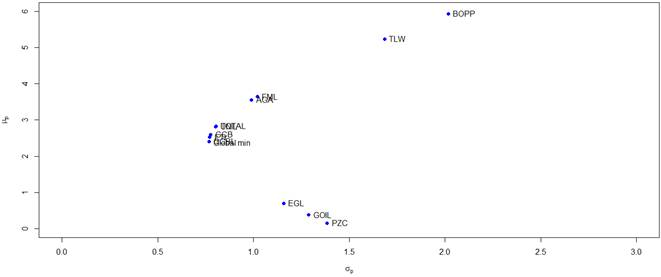Figure 2. Efficient Frontier.

4. Conclusion

The purpose of this paper is to construct optimal portfolio using matrix approach. The results indicate that, all the portfolios are optimal and that portfolios 1, 2, 4, 5, 6, 9, 10, 11 and 12 with expected return 2.523, 2.593, 2.827, 3.642, 2.405, 2.812, 5.229, 3.559 and 5.928 respectively are efficient portfolios whereas portfolios 3, 7 and 8 with expected return 0.377, 0.699 and 0.152 respectively are inefficient portfolios with reference to the expected return of the global minimum variance portfolio (2.360). GGBL is seen as the stock with the highest allocation of wealth in most of the portfolios. Six out of the 12 portfolios had CLYD exhibiting the least asset allocation. It is therefore advisable for investors to consider investing in the efficient portfolios by taking into consideration the weight of each portfolio so as to minimize risk in other to get the desired return. Also, it is seen that investing in only one asset bares a higher risk than investing in several assets hence the need for investors to diversify their portfolios.

References

1. Bawa V.S., Brown S.J. and Klein R.W (1979). Estimation Risk and Optimal Portfolio Choice. North-Holland. Amsterdam, the Netherland.
2. Ceria, S. and Stubbs, R.A (2006). Incorporating estimation errors into portfolio selection: Robust portfolio construction. Journal of Asset Management 7(2): 109-127.
3. Costa, O.L.V. and Paiva, A.C (2002). Robust Portfolio Selection Using Linear-matrix Inequality. Journal of Economic Dynamics and Control 26:889-909.
4. Drake, P.P and Fabozzi, F.J (2010). The Basics of Finance: An Introduction to Financial Markets, Business Finance and Portfolio Management. John Wiley and Sons, New Jersy.
5. Frantz, P. and Payne, R. (2009). Corporate finance. Chapter 2. London: University of London Press.
6. Cornuejols, G. and Tutuncu, R.(2007): Optimization methods in Finance. Cambridge University Press, Cambridge.
7. Goldfarb, D. and Lyenga,r G. (2003): Robust portfolio selection problems. Math. Opr. Res. 28:1-38.
8. Jagannathan, R. and Ma, T. (2003). "Risk Reduction in Large Portfolios: Why Imposing the Wrong Constraints Helps". Journal of Finance 58: 1651-1684.
9. Manuel Tarrazo (2013). Portfolio Optimization with Correlation Matrix: How, Why, and Why Not. Journal of Finance and Investment Analysis 2(3):17-34.
10. Markowitz, H.M (1952). Portfolio Selection. Journal of finance 7:77-91.
11. Markowitz, H.M (1959). Portfolio selection Efficient Diversification of Investments, Wiley, New York.
12. Merton, R. C. (1980). On Estimation of Expected Return on the Market: "An Exploratory Investigation". Journal of Financial Economics 8:323-361.
13. Mulvey, J. and Erkan H.G (2003). Decentralized Risk Management for Global P/C Insurance Companies. Working paper.In: Applications of stochastic programming. Eds:Wallace S.W., Ziemba W.T. MPS-SLAM Series on optimization, Philadephia, PA,503-530.
14. Myles E. M (2013). A Simplified Perspective ofthe Markowitz Portfolio Theory. Global Journal of Business Research 7(1): 59-70
15. Schneeweis, T., Crowder, G., Kazemi, H., (2010). The new science of asset allocation. Risk management in a multi-asset world. Hoboken, NJ: Wiley & Sons.
16. Tutuncu, R.H. and Koeing M.: Robust asset allocation. Annal of Operations Research 132:157-187.

 Contents 1. 2. 2.1. 2.2. 2.3. 2.4. 2.5. 3. 4.
Article ToolsAbstractPDF(288K)